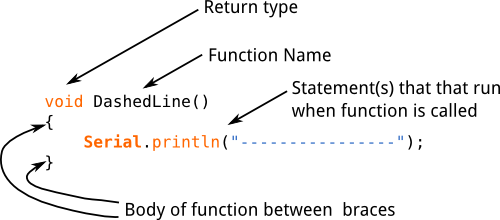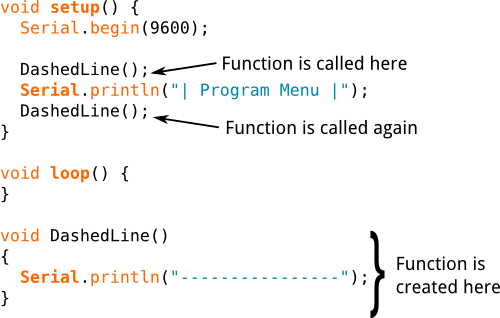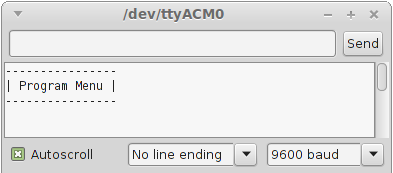Starting Electronics needs your help! Please make a donation to help cover our hosting and other costs. Click the donate button to send a donation of any amount.# Arduino Functions

Created on: 4 March 2015

Part 15 of the Arduino Programming Course

In this part of the Arduino programming course, you will learn how to write your own functions and use them in your sketches. The structure and use of functions is fully explained.

Functions were briefly encountered in part 1 of this programming course where some basic facts about functions where stated – 1) each function must have a unique name, 2) the function name is followed by parentheses () 3) functions have a return type, e.g. void, 4) the body of a function is enclosed in opening and closing braces {}.

We will start by examining the structure of a function and then see how to write functions.

## The Structure of a Function

Before a function can be used in a sketch, it must be created. The following code is an example of a function that was created to print a dashed line in the Arduino IDE.

```void DashedLine()
{
Serial.println("----------------");
}
```

The code above that creates the function is called the function definition. The image below shows the components of a function.Structure of a Simple Arduino Function

### Function Name

When we create a function, it must be given a name. The naming convention for functions is the same as for variables:

• The function name can be made up of alphanumeric characters (A to Z; a to z; 0 to 9) and the underscore (_).
• The function name may not start with a number i.e. the numbers 0 to 9.
• A function name must not be used that is the same as a language keyword or existing function.

The function name ends with parentheses (). Nothing is passed to the example function above, so the parentheses are empty. Passing values or parameters to functions will be explained later in this tutorial.

### Return Type

A function must have a return type. The example function does not return anything, so has a return type of void. Returning a value from a function will be explained in the next part of this course.

### Function Body

The function body is made up of statements placed between braces {}. The statements make up the functionality of the function (what the function will do when it is called).

When a function is used, it is said to be "called". We will look at how to call a function next.

## Calling a Function

To use the function that was created above, it must be called in a sketch as shown in the sketch below.

```void setup() {
Serial.begin(9600);

DashedLine();
DashedLine();
}

void loop() {
}

void DashedLine()
{
Serial.println("----------------");
}

```

In the sketch above, the DashedLine() function is created at the bottom of the file and then called twice at the top of the file as shown in the image below.Calling a Function in an Arduino Sketch

To call a function, use the function name followed by opening and closing parentheses. Finally terminate the statement that calls the function with a semicolon.

Load the sketch to an Arduino and then open the terminal window. The sketch prints some text in a box as shown below.Output from the DashedLine() Function

The first time that the function is called, it prints the dashed line shown in the top of the image. Text is then written to the serial monitor window by the statement below the function call. The function is then called again to print the same dashed line that completes the box.

## Why Use Functions

The function used in the example above is very simple, so all the benefits of using functions will not be seen immediately.

One advantage of using functions is that they avoid having to write the same code over and over again in a sketch which saves time and memory. Every time that a function is called, we are just reusing code that has been written once.

If a function needs to be modified, it only has to be done once and the modifications will take effect every place in a sketch that the function is called. If a function was not used, each place that the statements are found in a sketch to do a particular task would need to be located and modified.

Functions can be used to break a sketch up into pieces which make it more modular and easier to understand. Functions can be reused in other sketches.

## Passing a Value to a Function

In the sketch above, the length of the line that the function prints out is fixed in the function. If we change the text that is in the box, it may not fit in the box properly. The function needs to be modified so that we can tell it what size line it must draw.

The above function can be modified to pass a value to it that will tell it how many characters long to make the line that it draws.

The modified sketch is shown below.

```void setup() {
Serial.begin(9600);

DashedLine(24);
DashedLine(24);
}

void loop() {
}

void DashedLine(int len)
{
int i;

// draw the line
for (i = 0; i < len; i++) {
Serial.print("-");
}
// move the cursor to the next line
Serial.println("");
}

```

This video shows the modified sketch running:

Can't see the video? View on YouTube →

The DashedLine() function in the above sketch is modified so that an integer value can be passed to it. The line needs to be 24 characters long to fit the new menu text into it, so we pass it a value of 24.

```DashedLine(24);  // passing a value of 24 to the function
```

Of course the function has to be modified to handle the value that is being passed to it:

```void DashedLine(int len)
{
int i;

// draw the line
for (i = 0; i < len; i++) {
Serial.print("-");
}
// move the cursor to the next line
Serial.println("");
}
```

The function needs to be able to accept an integer value that is passed to it. The variable type and the name of the variable are inserted between the opening an closing parentheses after the function name.

```void DashedLine(int len)
```

We can now use the len integer variable in the body of the DashedLine() function. The variable will contain whatever value was passed to it when the function was called.

The body of the sketch uses the len variable in a for loop to print out the correct number of dashes that make up the dashed line of the menu box.

The cursor is moved to the next line in the serial monitor by calling Serial.println(""); with an empty string.

After the function has run the last statement in its body, it is said to "return". When a function returns, program execution continues below the statement that called the function – i.e. the statement below the function call is run next.